One end of a long metallic wire of length L is tied to the ceiling. The other end is tied to massless spring of spring constant K. A mass m hangs freely from the free end of the spring. The area of cross-section and Young's modulus of the wire is A and Y respectively. If the mass is slightly pulled down and released, it will oscillate with a time period T equal to -

(a)    $2\mathrm{\pi }\left(\frac{\mathrm{m}}{\mathrm{K}}\right)$                   (b)     $2\mathrm{\pi }{\left\{\frac{\left(\mathrm{YA}+\mathrm{KL}\right)\mathrm{m}}{\mathrm{YAK}}\right\}}^{1/2}$

(c)    $2\mathrm{\pi }\frac{\mathrm{mYA}}{\mathrm{KL}}$                (d)      $2\mathrm{\pi }\frac{\mathrm{mL}}{\mathrm{YA}}$

Concept Questions :-

Combination of springs
High Yielding Test Series + Question Bank - NEET 2020

Difficulty Level:

On a smooth inclined plane, a body of mass M is attached between two springs. The other ends of the springs are fixed to firm supports. If each spring has force constant K, the period of oscillation of the body (assuming the springs as massless) is

(a) $2\mathrm{\pi }{\left(\frac{M}{2K}\right)}^{1/2}$           (b) $2\mathrm{\pi }{\left(\frac{2\mathrm{M}}{K}\right)}^{1/2}$

(c)            (d) $2\mathrm{\pi }{\left(\frac{2\mathrm{Mg}}{\mathrm{K}}\right)}^{1/2}$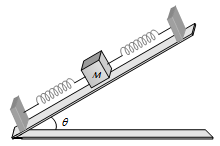Concept Questions :-

Combination of springs
High Yielding Test Series + Question Bank - NEET 2020

Difficulty Level:

A particle of mass m is attached to a spring (of spring constant k) and has a natural angular frequency ${\omega }_{0}$. An external force F (t) proportional to  is applied to the oscillator. The time displacement of the oscillator will be proportional to -

(a) $\frac{m}{{{\omega }^{2}}_{0}-{\omega }^{2}}$                 (b) $\frac{1}{m\left({{\omega }^{2}}_{0}-{\omega }^{2}\right)}$

(c) $\frac{1}{m\left({{\omega }^{2}}_{1}+{\omega }^{2}\right)}$            (d) $\frac{m}{{{\omega }^{2}}_{1}+{\omega }^{2}}$

Concept Questions :-

Forced oscillation
High Yielding Test Series + Question Bank - NEET 2020

Difficulty Level:

An ideal spring with spring-constant K is hung from the ceiling and a block of mass M is attached to its lower end. The mass is released with the spring initially unstretched. Then the maximum extension in the spring is -

(a) 4 Mg/K          (b) 2 Mg/K

(c) Mg/K             (d) Mg/2K

Concept Questions :-

Combination of springs
High Yielding Test Series + Question Bank - NEET 2020

Difficulty Level:

The displacement y of a particle executing periodic motion is given by $y=4{\mathrm{cos}}^{2}\left(t/2\right)\mathrm{sin}\left(1000t\right).$ This expression may be considered to be a result of the superposition of  ........... independent harmonic motions

1. Two

2. Three

3. Four

4. Five

Concept Questions :-

Simple harmonic motion
High Yielding Test Series + Question Bank - NEET 2020

Difficulty Level:

Three masses 700g, 500g, and 400g are suspended at the end of a spring as shown and are in equilibrium. When the 700g mass is removed, the system oscillates with a period of 3 seconds. When the 500 gm mass is also removed, it will oscillate with a period of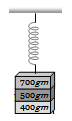1. 1 s

2. 2 s

3. 3 s

4. $\sqrt{\frac{12}{5}}s$

Concept Questions :-

Combination of springs
High Yielding Test Series + Question Bank - NEET 2020

Difficulty Level:

A particle of mass m is attached to three identical springs A, B and C each of force constant k a shown in figure. If the particle of mass m is pushed slightly against the spring A and released then the time period of oscillations is -

(a) $2\mathrm{\pi }\sqrt{\frac{2\mathrm{m}}{\mathrm{k}}}$          (b) $2\mathrm{\pi }\sqrt{\frac{\mathrm{m}}{2\mathrm{k}}}$

(c) $2\mathrm{\pi }\sqrt{\frac{\mathrm{m}}{\mathrm{k}}}$            (d) $2\mathrm{\pi }\sqrt{\frac{\mathrm{m}}{3\mathrm{k}}}$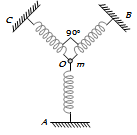Concept Questions :-

Combination of springs
High Yielding Test Series + Question Bank - NEET 2020

Difficulty Level:

The graph shows the variation of displacement of a particle executing S.H.M. with time. We infer from this graph that -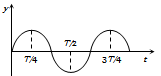(a) The force is zero at time T/8

(b) The velocity is maximum at time T/4

(c) The acceleration is maximum at time T

(d) The P.E. is equal to total energy at time T/4

Concept Questions :-

Energy of SHM
High Yielding Test Series + Question Bank - NEET 2020

Difficulty Level:

A particle of mass m oscillates with simple harmonic motion between points ${x}_{1}$ and ${x}_{2}$ , the equilibrium position being O. Its potential energy is plotted. It will be as given below in the graph

1.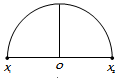2.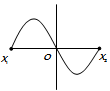3.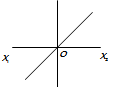4.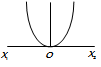Concept Questions :-

Energy of SHM
High Yielding Test Series + Question Bank - NEET 2020

Difficulty Level:

For a particle executing S.H.M. the displacement x is given by . Identify the graph which represents the variation of potential energy (P.E.) as a function of time t and displacement x.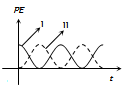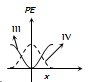(a) I, III          (b) II, IV

(c) II, III         (d) I, IV

Concept Questions :-

Energy of SHM